Tuesday, 05 April 2011 07:42

### PDF Graphics Transparency in C#, VB.NET

The sample shows the different modes of transparency.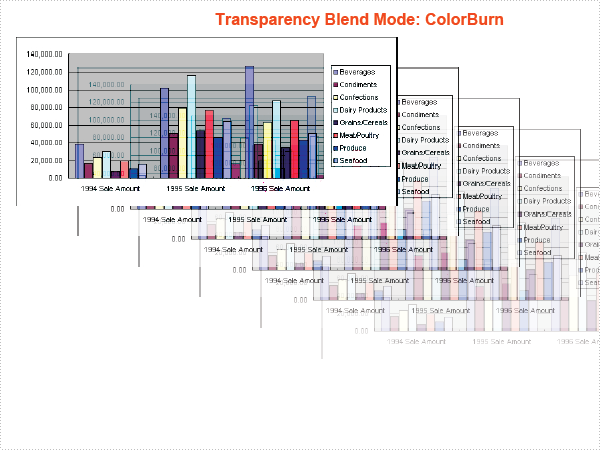Tuesday, 05 April 2011 07:27

### PDF Barcode in C#, VB.NET

The sample demonstrates how to draw barcode in PDF document.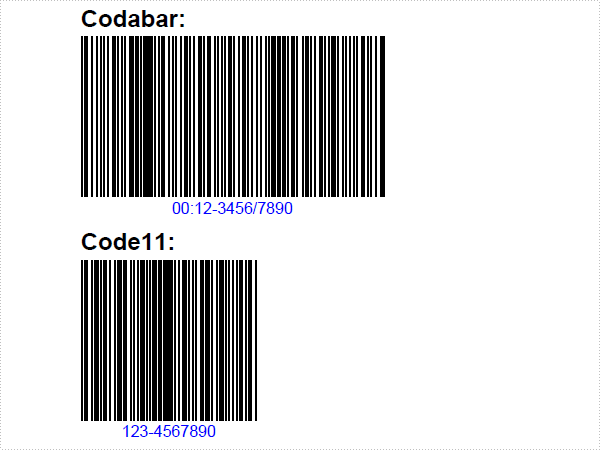Tuesday, 05 April 2011 07:21

### PDF DrawImage in C#, VB.NET

The sample demonstrates how to draw image in PDF document.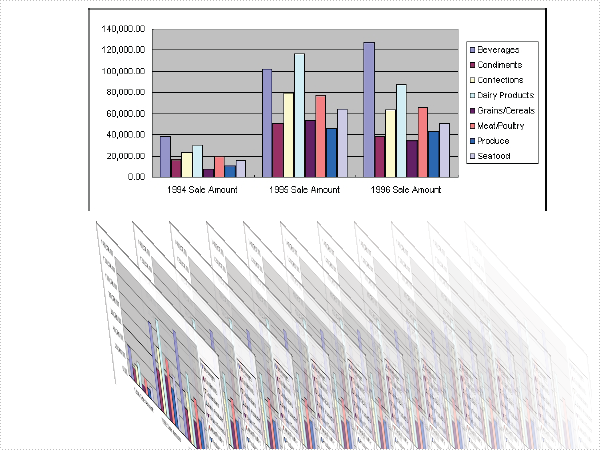Tuesday, 05 April 2011 07:19

### PDF DrawShape in C#, VB.NET

The sample demonstrates how to draw shape to a PDF document.Tuesday, 05 April 2011 07:12

### PDF DrawText in C#, VB.NET

The sample demonstrates how to draw text to a PDF document.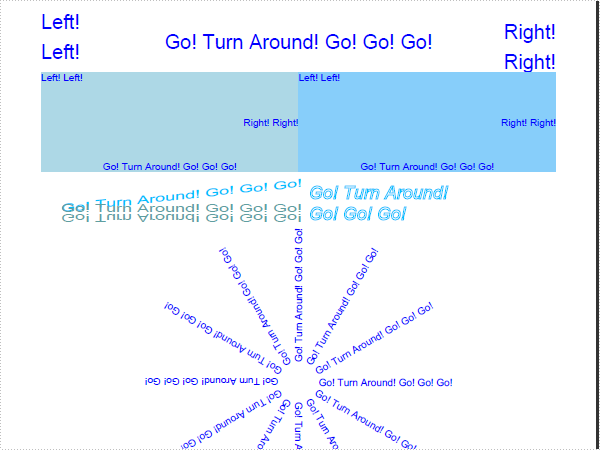Tuesday, 05 April 2011 06:47

### PDF Image in C#, VB.NET

The sample demonstrates how to insert an image to a PDF document.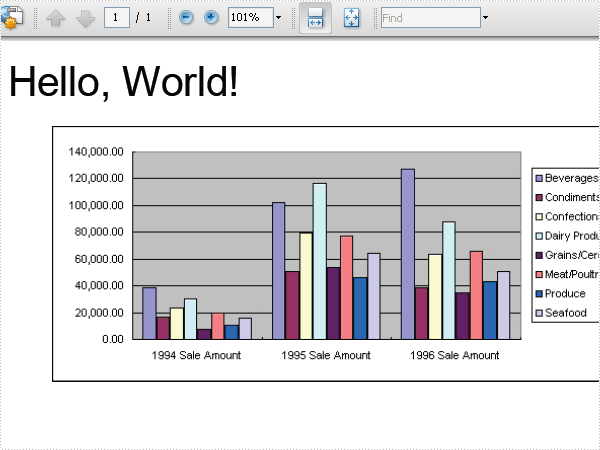Friday, 01 April 2011 08:52

### PDF HelloWorld in C#, VB.NET

The sample demonstrates how to write a "HelloWorld" to a PDF document.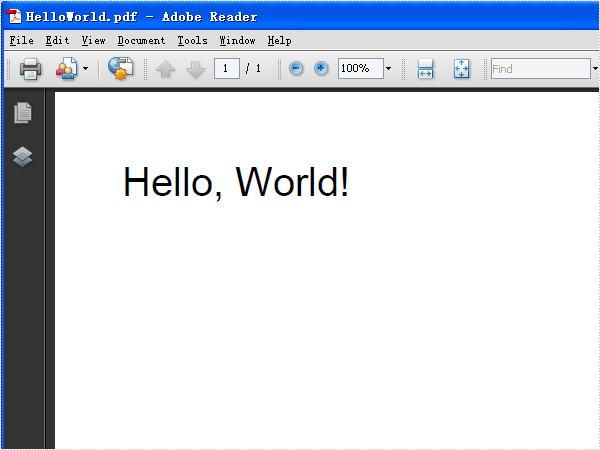Monday, 24 January 2011 09:52

### Generate Excel Column Chart in C#, VB.NET

Excel Charts uses graphics to present data information to enable users to analyze data more intuitively. According to details of data information, users need to choose the most appropriate chart type. For example, column chart is suitable for comparison between data, while pie chart is often used to show percentage of each item among all.

Spire.XLS for .NET, a stand-alone Excel operation component designed for .NET developers, allows users to generate Excel chart, and this guide will show you how to generate excel column chart with C#, VB.NET via Spire.XLS for .NET. Users can use sheet.Charts.Add(ExcelChartType) method to add chart in Excel. Then, assign value for DataRange property of Chart class to fill information in chart area. Next, set position, chart title, axes, legend and format them for chart to make the chart be more completed and wonderful.

The following screenshot shows the result of Excel column chart generation.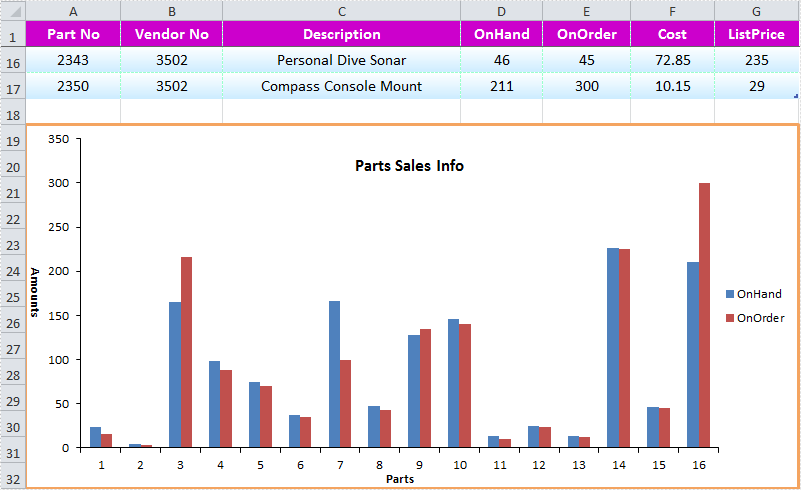Download and install Spire.XLS for .NET. Use the following code to generate Excel column chart.

[C#]
```using System.Drawing;
using Spire.Xls;

namespace XLSTest
{
class Program
{
static void Main(string[] args)
{
Workbook workbook = new Workbook();
Worksheet sheet = workbook.Worksheets;

//Add Chart and Set Chart Data Range
chart.DataRange = sheet.Range["D1:E17"];
chart.SeriesDataFromRange = false;

//Chart Position
chart.LeftColumn = 1;
chart.TopRow = 19;
chart.RightColumn = 8;
chart.BottomRow = 33;

//Chart Border
chart.ChartArea.Border.Weight = ChartLineWeightType.Medium;
chart.ChartArea.Border.Color = Color.SandyBrown;

//Chart Title
chart.ChartTitle = "Parts Sales Info";
chart.ChartTitleArea.Font.FontName = "Calibri";
chart.ChartTitleArea.Font.Size = 13;
chart.ChartTitleArea.Font.IsBold = true;

//Chart Axes
chart.PrimaryCategoryAxis.Title = "Parts";
chart.PrimaryCategoryAxis.Font.Color = Color.Blue;

chart.PrimaryValueAxis.Title = "Amounts";
chart.PrimaryValueAxis.HasMajorGridLines = false;
chart.PrimaryValueAxis.MaxValue = 350;
chart.PrimaryValueAxis.TitleArea.TextRotationAngle = 90;

//Chart Legend
chart.Legend.Position = LegendPositionType.Right;

//Save and Launch
workbook.SaveToFile("ExcelColumnChart.xlsx",ExcelVersion.Version2010);
System.Diagnostics.Process.Start("ExcelColumnChart.xlsx");

}
}
}
```
[VB.NET]
```Imports System.Drawing
Imports Spire.Xls

Namespace XLSTest
Friend Class Program
Shared Sub Main(ByVal args() As String)
Dim workbook As New Workbook()
Dim sheet As Worksheet = workbook.Worksheets(0)

'Add Chart and Set Chart Data Range
Dim chart As Chart = sheet.Charts.Add(ExcelChartType.ColumnClustered)
chart.DataRange = sheet.Range("D1:E17")
chart.SeriesDataFromRange = False

'Chart Position
chart.LeftColumn = 1
chart.TopRow = 19
chart.RightColumn = 8
chart.BottomRow = 33

'Chart Border
chart.ChartArea.Border.Weight = ChartLineWeightType.Medium
chart.ChartArea.Border.Color = Color.SandyBrown

'Chart Title
chart.ChartTitle = "Parts Sales Info"
chart.ChartTitleArea.Font.FontName = "Calibri"
chart.ChartTitleArea.Font.Size = 13
chart.ChartTitleArea.Font.IsBold = True

'Chart Axes
chart.PrimaryCategoryAxis.Title = "Parts"
chart.PrimaryCategoryAxis.Font.Color = Color.Blue

chart.PrimaryValueAxis.Title = "Amounts"
chart.PrimaryValueAxis.HasMajorGridLines = False
chart.PrimaryValueAxis.MaxValue = 350
chart.PrimaryValueAxis.TitleArea.TextRotationAngle = 90

'Chart Legend
chart.Legend.Position = LegendPositionType.Right

'Save and Launch
workbook.SaveToFile("ExcelColumnChart.xlsx", ExcelVersion.Version2010)
System.Diagnostics.Process.Start("ExcelColumnChart.xlsx")

End Sub
End Class
End Namespace
```
Monday, 24 January 2011 09:49

### Save Excel Document in C#, VB.NET

Automation of an Excel file allows us to doing various operations in C#/VB.NET. Any loss in these operations may result in unexpected negative consequences for developers and the clients of the developers. That means we must find a solution that enables us to Save Excel with no loss in quality of our operations. This section will demonstrate how to fast save Excel file with perfect performance as directly operations in Excel files.

Spire.Xls for .NET is a professional component that enables developers directly manages Excel operation regardless whether Microsoft Excel is installed on or not. With Spire.Xls for .NET, we can save Excel to what we want it to be. Any kind of trial and evaluation on Spire.Xls for .NET is always welcomed; so now please feel free to download Spire.Xls for .NET and then follow our guide to save perfect Excel or try other function of Spire.Xls for .NET.

Spire.Xls for .NET allows us to create a new Excel file, write data in to it, edit the input data and then save Excel file.

[C#]
```using Spire.Xls;
namespace Excel_save
{
class Program
{
static void Main(string[] args)
{
//Create a new workbook
Workbook workbook = new Workbook();
//Initialize worksheet
Worksheet sheet = workbook.Worksheets;
//Append text
sheet.Range["A1"].Text = "Demo: Save Excel in .NET";
//Save it as Excel file
workbook.SaveToFile("Sample.xls",ExcelVersion.Version97to2003);
//Launch the file
System.Diagnostics.Process.Start(workbook.FileName);
}
}
}
```
[VB.NET]
```Imports Spire.Xls
Namespace Excel_save
Class Program
Private Shared Sub Main(args As String())
'Create a new workbook
Dim workbook As New Workbook()
'Initialize worksheet
Dim sheet As Worksheet = workbook.Worksheets(0)
'Append text
sheet.Range("A1").Text = "Demo: Save Excel in .NET"
'Save it as Excel file
workbook.SaveToFile("Sample.xls",ExcelVersion.Version97to2003)
'Launch the file
System.Diagnostics.Process.Start(workbook.FileName)
End Sub
End Class
End Namespace
```
Monday, 24 January 2011 09:43

### Add Excel Formulas in C#, VB.NET

In an Excel Worksheet, we may import a great deal of data. Sometimes, we need to calculate the data to get other numbers we need. It is a time consuming job when we deal with large spreadsheets and more complex data. Formula is the main tool to calculate data. There are various formulas included in Excel. Below I will show you how to add formula in C#, VB.NET by Spire.XLS.

Spire.XLS .NET is a Professional and stable .NET Excel component which enables developers/programmers to operate Excel files with their ASP.NET web sites and Windows Forms applications. It supports calculate complex Excel Formulas. Spire.XLS presents you an easiest way to add formula for Excel.

Please check the effective screenshot first.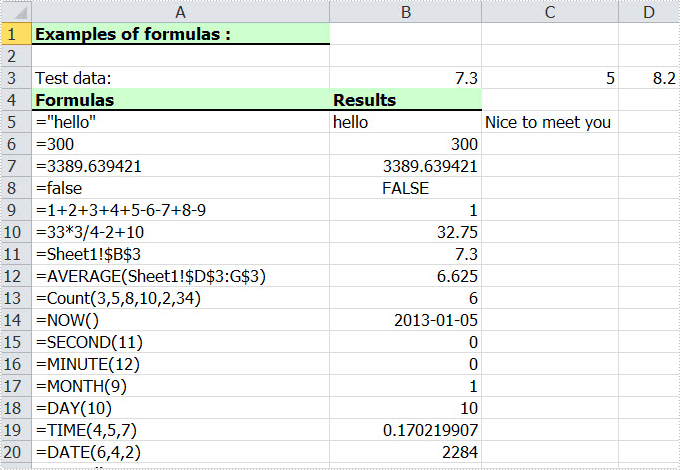Now we will give you a demo with many kinds of formulas written in the worksheet. The formula can be string, bool value, calculation, sheet area reference, time and so on. Here, we first reference a variable "currentRow" to control rows of all kinds of formulas. Second, reference "currentFormula". Assign value for Worksheet.Range [cellRange]. Text to write a formula, and then via currentFormula to assign value for Worksheet.Range [cellRange]. Formula to get the formula results.

[C#]
```using Spire.Xls;

namespace Formula
{
class Program
{
static void Main(string[] args)
{
//Create a new workbook
Workbook workbook = new Workbook();

//Initialize worksheet
Worksheet sheet = workbook.Worksheets;

//initialize currentRow
int currentRow = 3;
string currentFormula = string.Empty;

//test data
sheet.Range[currentRow, 2].NumberValue = 7.3;
sheet.Range[currentRow, 3].NumberValue = 5; ;
sheet.Range[currentRow, 4].NumberValue = 8.2;
sheet.Range[currentRow, 5].NumberValue = 4;
sheet.Range[currentRow, 6].NumberValue = 3;
sheet.Range[currentRow, 7].NumberValue = 11.3;

//string.
currentFormula = "=\"hello\"";
sheet.Range[++currentRow, 1].Text = "=\"hello\"";
sheet.Range[currentRow, 2].Formula = currentFormula;

//bool.
currentFormula = "=false";
sheet.Range[++currentRow, 1].Text = currentFormula;
sheet.Range[currentRow, 2].Formula = currentFormula;

//calculation
currentFormula = "=33*3/4-2+10";
sheet.Range[++currentRow, 1].Text = currentFormula;
sheet.Range[currentRow, 2].Formula = currentFormula;

//sheet area reference
currentFormula = "=AVERAGE(Sheet1!\$D\$3:G\$3)";
sheet.Range[++currentRow, 1].Text = currentFormula;
sheet.Range[currentRow, 2].Formula = currentFormula;

//time
currentFormula = "=NOW()";
sheet.Range[++currentRow, 1].Text = currentFormula;
sheet.Range[currentRow, 2].Formula = currentFormula;
sheet.Range[currentRow, 2].Style.NumberFormat = "yyyy-MM-DD";

//Save the file
workbook.SaveToFile("Sample.xls",ExcelVersion.Version97to2003);

//Launch the file
System.Diagnostics.Process.Start("Sample.xls");
}
}
}
```
[VB.NET]
```Imports Spire.Xls

Module Module1

Sub Main()
'Create a new workbook
Dim workbook As New Workbook()

'Initialize worksheet
Dim sheet As Worksheet = workbook.Worksheets(0)

'initialize currentRow
Dim currentRow As Integer = 3
Dim currentFormula As String = String.Empty

'test data
sheet.Range(currentRow, 2).NumberValue = 7.3
sheet.Range(currentRow, 3).NumberValue = 5

sheet.Range(currentRow, 4).NumberValue = 8.2
sheet.Range(currentRow, 5).NumberValue = 4
sheet.Range(currentRow, 6).NumberValue = 3
sheet.Range(currentRow, 7).NumberValue = 11.3

'string.
currentFormula = "=""hello"""
sheet.Range(currentRow, 2).Formula = currentFormula

'bool.
currentFormula = "=false"
sheet.Range(currentRow, 2).Formula = currentFormula

'calculation
currentFormula = "=33*3/4-2+10"
sheet.Range(currentRow, 2).Formula = currentFormula

'sheet area reference
currentFormula = "=AVERAGE(Sheet1!\$D\$3:G\$3)"
sheet.Range(currentRow, 2).Formula = currentFormula

'time
currentFormula = "=NOW()"
sheet.Range(currentRow, 2).Formula = currentFormula
sheet.Range(currentRow, 2).Style.NumberFormat = "yyyy-MM-DD"

'Save doc file.
workbook.SaveToFile("Sample.xls",ExcelVersion.Version97to2003)

'Launching the MS Word file.
System.Diagnostics.Process.Start("Sample.xls")

End Sub
End Module
```

Spire.XLS supports many methods to add a formula for Excel including different parts. As a professional .NET Excel component, it owns the ability of inserting content into Excel document, formatting cells and converting Excel documents to popular office file formats. Spire.XLS for WPF supports Excel 97-2003, Excel 2007 and Excel 2010.

Page 10 of 22